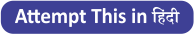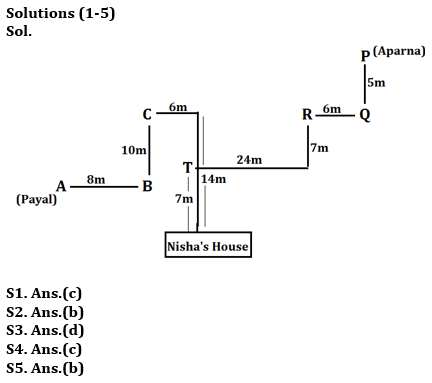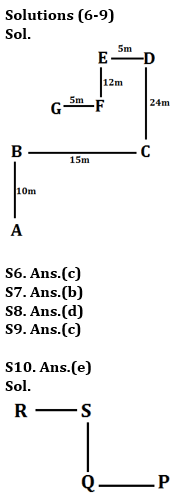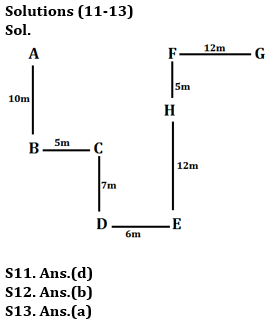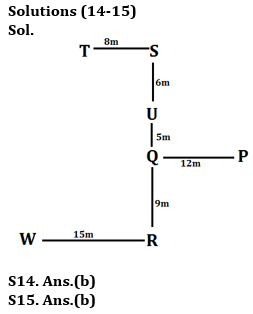Latest Banking jobs   »   Reasoning Ability Quiz For IBPS RRB...

# Reasoning Ability Quiz For IBPS RRB PO, Clerk Prelims 2021- 4th June

Directions (1-5): Study the following information carefully and answer the questions given below:
Payal starts walking from point A, walks 8m in east direction and reach at point B. From point B she takes a left turn and walks 10m to reach at point C, then takes two consecutive right turn and walks 6m and 14m respectively to reach her friend Nisha’s house. Aparna starts walking in south direction from point P and walks 5m to reach at point Q, then takes a right turn and walks 6m to reach at point R. From point R she takes a left turn and walks 7m to reach at point S. From point S she takes a right turn and walks 24m and reach at point T, then finally takes a left turn and walks 7m to reach her friend Nisha’s house.

Q1. What is the distance between point C to point R?
(a) 12m
(b) 28m
(c) 30m
(d) 20m
(e) None of these

Q2. What is the shortest distance between point S to Nisha’s house?
(a) 49m
(b) 25m
(c) 16m
(d) 30m
(e) None of these

Q3. What is the direction of point P with respect to point B?
(a) South-West
(b) North
(c) East
(d) North-East
(e) None of these

Q4. What is the direction and distance between point T with respect to point B?
(a) 3√5m, south-west
(b) 45m, east
(c) 3√5m, north-east
(d) 35, west
(e) None of these

Q5. What is the direction of point T with respect to point P?
(a) South
(b) South-West
(c) North-East
(d) West
(e) None of these

Directions (6-9): Study the information carefully and answer the questions given below.
Point B is 10m north of A. Point C is 24m south of D which is 5m east of E. Point G is 5m west of F. Point C is 15m east of B. point F is 12m south of point E.

Q6. What is the shortest distance between G and B?
(a) 14m
(b) 11m
(c) 13m
(d)10m
(e) 5m

Q7. What is the direction of E with respect of G?
(a) South-west
(b) North-east
(c) South
(d) North
(e) North-west

Q8. If point H is 8m north of C, what is the direction and distance of Point H with respect to B?
(a) 12m, North-East
(b) 7m, North
(c) 15m, South-west
(d) 17m, North-East
(e) 10m, North-west

Q9. What is the direction of A with respect to F?
(a) South- East
(b) North- West
(c) South-West
(d) North
(e) South

Q10. If A@B means ‘B is west of A’, A#B means ‘A is south of B’, A%B means ‘A is east of B’ and A&B means ‘A is north of B’. Then what is the direction of P with respect of R in the given expression- P % Q # S @ R
(a) North
(b) South
(c) North- East
(d) South- West
(e) South- East

Point D is 7m south of Point C which is 5m east of B. Point F is 12m west of G. Point E is 6m east of D and Point A is 10m north of B. Point E is 17m south of F and Point H 12m north of E.

Q11. What is the shortest distance between point H and G?
(a) 10m
(b) 25m
(c) 16m
(d) 13m
(e) 7m

Q12. What is the direction of point E with respect to A?
(a) South
(b) South-East
(c) South- West
(d) North-West
(e) North

Q13. If point P is 5m west of point D, then what is the distance and direction of point P with respect to A?
(a) 17m, South
(b) 12m, South-West
(c) 10m, North
(d) 15m, West
(e) 11m, North-east

Directions (14-15): Study the following information carefully and answer the given questions:
Point P is 12m east of point Q. Point Q is 9m north of point R and also 11m south of point S. Point T is 8m west of point S. Point R is 14m south of point U. Point W is 15m west of point R.

Q14. Point W is in which direction with respect to point S?
(a) North-west
(b) South-west
(c) North-east
(d) South-east
(e) None of these

Q15. What is the shortest distance between U and P?
(a) √29m
(b) 13m
(c) √37m
(d) 6m
(e) None of these

Practice More Questions of Reasoning for Competitive Exams:

###### Study Plan for IBPS RRB PO/Clerk Prelims 2021

Solutions